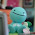## Monday, January 17, 2011

### Final Percent Post

4.1 Representing Percents : When representing percents , there's many ways you can show your percent . The easiest way is through a 100 grid . Mostly because a percent is out of 100 .

Example : 80/100 . To represent that you draw a 100 grid and shade in 80 Squares .

4.2 Fractions , Decimals & Percents : This is when you use various ways to convert Percents to fractions or decimals . There are many ways you could do this . One way you could find percent is by Multiplying or Dividing a number by 100 . To find the percent out of 100 .

Example : 30% of 100 . 30/100 = 0.3 0.3x100= 30

4.3 Percent of a number : Where you find a percent out of any number , It could be more than 100 or less that 1% .

Example : 3/4% = 0.75 . 150% of \$10 = \$15.00

4.4 Combining Percents : This is where you add to a Percent . As in , adding more to the additional percent . Having more than the regular percentage .

Example : Tax in Canada = 12% . If something cost \$10.00 .

10.00x1.12=\$11.20

#### 1 comment:

1.You should've added some colour and some bolded or italicized wording so that the important parts would pop out more. I also like how you used examples, great job Lara. (: